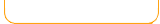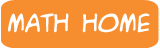Newly Converted games - works on tablets & phones!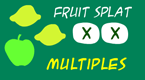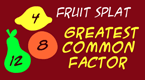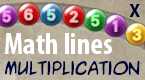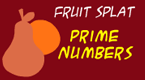Keep checking back - more games added all the time!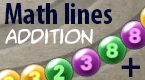What is a factor? Factors are numbers that are multiplied together to get another number. When you factor a number, you are taking the numbers apart and finding the indiviual numbers (factors) that are multiplied within it. Example: What are the factors of the number 6? They are 2 and 3, because 2 x 3 = 6. Also, factors of 6 are 6 and 1, because 6 x 1 = 6. Note: All numbers have the factor of 1, because 1 multiplied by a number equals the same number. So, the factors of 6 are: 1, 2, 3, 6. Example: What are the factors of the number 12? What numbers can be multiplied to get 12? 3 x 4 = 12 2 x 6 = 12 1 x 12 - 12 So the factors of 12 are 1, 2, 3, 4, 6, 12 Example: What are the factors for the number 16? 1 x 16 = 16 2 x 8 = 16 4 x 4 = 16 So the factors of 16 are 1, 2, 4, 8, 16 Prime Numbers A prime number has exactly two factors - the number 1 and itself. It does not have any other factors. Here are some example of prime numbers: Example: the number 7 The only numbers that can divide evenly into 7 are 1 and 7. So 7 is a prime number. Other prime numbers: 2,3,5,7,11,13,17,19,23 ... and the list goes on.

Factors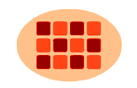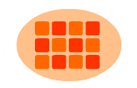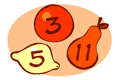Multiples Frenzy
2,3,4,5,6,7,8,9,10,11,12
Multiplication Station
Easy
Multiplication Station
Hard
Greatest Common Factor Least Common Multiple Prime Numbers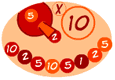Math Lines Math Lines Math Lines Math Lines Math Lines

 What is a factor? Factors are numbers that are multiplied together to get another number. When you factor a number, you are taking the numbers apart and finding the indiviual numbers (factors) that are multiplied within it. Example: What are the factors of the number 6? They are 2 and 3, because 2 x 3 = 6. Also, factors of 6 are 6 and 1, because 6 x 1 = 6. Note: All numbers have the factor of 1, because 1 multiplied by a number equals the same number. So, the factors of 6 are: 1, 2, 3, 6. Example: What are the factors of the number 12? What numbers can be multiplied to get 12? 3 x 4 = 12 2 x 6 = 12 1 x 12 - 12 So the factors of 12 are 1, 2, 3, 4, 6, 12 Example: What are the factors for the number 16? 1 x 16 = 16 2 x 8 = 16 4 x 4 = 16 So the factors of 16 are 1, 2, 4, 8, 16 Prime Numbers A prime number has exactly two factors - the number 1 and itself. It does not have any other factors. Here are some example of prime numbers: Example: the number 7 The only numbers that can divide evenly into 7 are 1 and 7. So 7 is a prime number. Other prime numbers: 2,3,5,7,11,13,17,19,23 ... and the list goes on.

 Learn math the fun way with our free educational games! With animation, music, and lots of fun- kids can enjoy practicing math and increase their math skills and math memory. Topics include: addition, subtraction, multiplication, division, fractions, decimals, numbers, geometry, shapes, early math, integers, time, measurement and much more! Game types involve puzzles, action games, arcade style, driving games, swimming, matching, memory and many more varieties for all kinds of learners and abilities. You can also find math videos and math game demonstrations at our math channel on youtube: